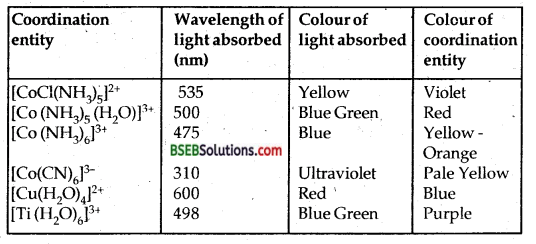# Bihar Board Class 12 Chemistry Solutions Chapter 9 Coordination Compounds

Bihar Board Class 12 Chemistry Solutions Chapter 9 Coordination Compounds Textbook Questions and Answers, Additional Important Questions, Notes.

## BSEB Bihar Board Class 12 Chemistry Solutions Chapter 9 Coordination Compounds

### Bihar Board Class 12 Chemistry Coordination Compounds Intext Questions and Answers

Question 1.
Write the formulae for the following coordination compounds.
(a) Tetraamminediaquacobalt (III) chloride
(b) Potassium tetracyanonitkelate (II).
(c)Tris (ethane-1,2-diamine) chromium (III) chloride.
(d) Amminebromidochloroidonitrito-N-platinate (II).
(e) Dichlorodobis (ethane-1,2-diamine) platinum (IV) nitrate
(f) Iron (III) hexacyanoferrate (II).
(a) [Co(NH3)4(H2O)2Cl3,
(b) K2[NI(CN)4]
(c) [Cr(en)3]Cl3
(d) [pt(NH3)Br Cl(NO2)]
(e) [ptCl2(en2)](NO3)2
(f) Fe4[Fe(CN)6]3

Question 2.
Write the IUPAC names of the following coordination compounds:
(a) [Co (NH3)6] Cl3
(b) [Co (NH3)5 Cl] Cl2,
(c) K3 [Fe (CN)6],
(d) K3 [Fe (C2O4)3],
(e) K2 [Pd Cl4],
(f) [Pt (NH3)2 Cl (NH2CH3)]Cl.
(a) Hexammine cobalt (III) chloride.
(b) Pentaamminechloridocobalt (III) chloride.
(c) Potassium hexacyanoferrate (III).
(d) .Potassium trioxalato ferrate (III).
(f) Diamminechlorido (methylamine) platinum (II) chloride.

Question 3.
Indicate the types of isomerism exihibited by the following complexes and draw the structures for these isomers:
(a) K[Cr(H2O)2(C2O4)2]
(b) [Co(en)3]Cl3
(c) [Co(NH3)5(NO2)](NO3)2
(d) [Pt(NH3)(H2O)Cl2].
(a) The complex [Cr(H2O)2(C2O4)2]+ shows geometrical isomerism; Cis and Transforms as shown below. Cis form shows optical isomerism.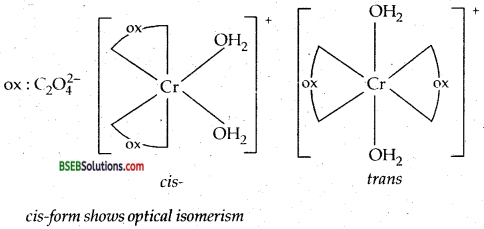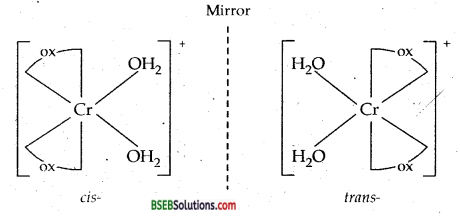(b) [CO(en)3]3+ exits in two optical isomers.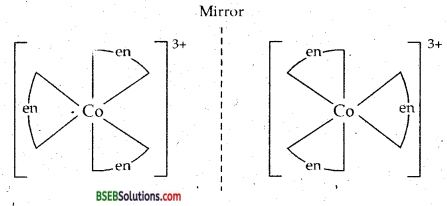(c) There are ten possible isomers. These are geometrical, ionisation and linkage isomers possible.
For example two ionisation isomers possible are [Co (NH3)5 (NO3)] (NO2)2 and [Co (NH3)5 (N02)] (N03)2 Two linkage isomers possible are :(d) Two geometrical isomers (Cis and trans) are possible.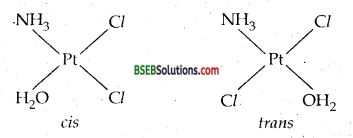Question 4.
Give evidence that [Co (NH3)5 Cl] SO4 and [Co (NH3)5 SO4] Cl are ionisation isomers.
The ionisation isomers dissolve in water to give different ions and thus react differently with various reagents:
[Co (NH3)5 Cl] SO4 + Ba2+ (aq) → BaSO4 (s) white ppt
[Co (NH3)5 SO4]Cl + Ba2+ (aq) → No reaction.
[Co (NH3)5 SO4]Cl + Ag+ (aq) → AgCl (s) white ppt
[CO(NH3)5Cl]SO4 + Ag+ (aq) → No reaction.

Question 5.
Explain on the basis of valence bond theory that [Ni (CN)4]2- ion with square planar structure is diamagnetic and the [Ni Cl4]2- ion with tetrahedral geometry is paramagnetic.
Ni = 28 = [Ar]3d8 4s2
Ni2+ or Ni (II) – [Ar] 3d8 4s0 or img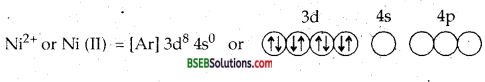Due to dsp2 hybridization the complex is square planar. Four CN ions deposit their four lone pairs in 4 dsp2 hybrid orbitais. Since it does not contain any unpaired electrons, hence [Ni (CN)4]2- is diamagnetic.Due to Sp3 hybridization [Ni Cl4]2- is tetrahedral. Since it contains two unpaired electrons in the inner two 3d orbitais hence the complex [Ni Cl4]2-paramagnetic.Question 6.
[Ni Cl4]2- is paramagnetic while [(Ni (CO)4] is diamagnetic though both are tetrahedral. Why?
[Ni Cl4]2- is tetrahedral and paramagnetic.Since it côntains two unpaired electrons, hence [Ni Cl4]2- is paramagnetic.
Oxidation state of nickel in the complex [(Ni (CO)4] is O. Its electronic configuration is [Ar] 3d84s2.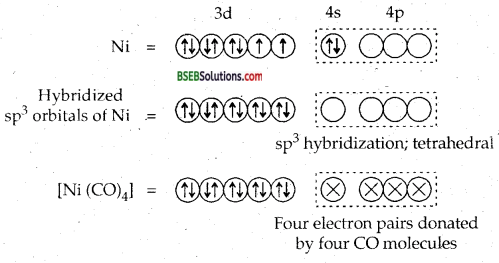Since it does not contain any unpaired electrons, it is diamagnetic. In the presence of CO ligand, the unpaired d electrons of Ni pair up but Cl being a weak ligand is unable to pair up the unpaired electrons.

Question 7.
[Fe (H2O)6]3+ is strongly paramagnetic whereas [Ee (CN)6FV is weakly paramagnetic. Explain.H2O is a weak ligand. Therefore, 3d electrons do not pair up. The hybridization is sp3d2 forming an outer orbital complex containing five unpaired electrons. Hence [Fe (H2O)6]3+ is strongly paramagnetic.In the presence of CN ions, (a strong ligand) the 3d electrons of Fe3+ ions pair up leaving only one unpaired electron in the 3d orbital as shown below:Hence [Fe (CN)6]3- is only weakly paramagnetic.

Question 8.
Explain [Co (NH3)6]3+ is an inner orbital complex whereas [Ni (NH3)6]2+ is an outer orbital complex.
In the presence of NH3 the 3d electrons pair up leaving two d- orbitals empty to be involved in d2sp3 hybridization forming inner orbital complex in [Co (NH3)6]3+. In (NH3)6]2+, Ni is in + 2 oxidation state and has d8 configuration, the hybridization involved is Sp3d2 forming outer orbital complex.

Question 9.
Predict the number of unpaired electrons in the square planar [Pt (CN)4]2- ion.
Since the complex [Pt (CN)4]2- is square planar. Therefore the hybridization is dsp2. Hence the unparied electrons in 5d orbitals pair up to make one d-orbital empty for dsp2 hybridization. Thus there is no unpaired electron.

Question 10.
The hexa quo manganese (II) ion contains five unpaired electrons, while the hexacyanOion contains only one unpaired electron. Explain using Crystal Field Theory.
[Mn (H2O)6]2+ has 5 unpaired electrons in 3d orbitals having one unpaired electron in each of the 3d orbitals because H2O is a weak ligand and crystal field splitting energy Δ0 < P, where P is the pairing energy. This energy is not sufficient to pair up the electrons in 3d orbitals.

[Mn (CN)6]4- on the other hand, has six-strong field CN ligands. Here Δ0 > P. The crystal field splitting energy being large forces the five electrons in 3d orbitals of manganese ion to pair up leaving only one unpaired electrons in 3d orbital.Question 11.
Calculate the overall complex dissociation equilibrium constant for the [Cu (NH3)4]2+ ion, given that β4 for this complex is 2.1 × 1013.
The overall dissociation constant is the reciprocal of overall stability constant, i.e.,$$\frac{1}{\beta_{4}}$$
∴ Overall dissociation constant = $$\frac{1}{2.1 \times 10^{13}}$$ = 4.7 × 10-14.

### Bihar Board Class 12 Chemistry The d-and f-Block Elements Text Book Questions and Answers

Question 1.
Explain the bonding in coordination compounds in terms of Werner’s postulates.
Werner’s Postulates for bonding in coordination compounds:
1. Every metal atom possesses two types of valencies (or linkages).

2.
(a) Primary linkage corresponds to the oxidation state of the metal atom.
(c) Primary linkages are satisfied with negative ions.

3.
(a) Secondary linkage corresponds to the coordination number of the metal atom.
(b) Secondary linkages are non-ionisable. The coordination number of a metal is a fixed number.
(c) Secondary linkages are satisfied either by negative ions or neutral ligands.
(d) Secondary linkages are directed towards fixed positions in space about the central metal atom or ion. As a result, the coordination compound acquires a definite shape or geometry. In the light of the postulates above, Werner, who had prepared the following compounds by reacting Cobalt (III) chloride with NH3, successfully explained the structure of these coordination compounds.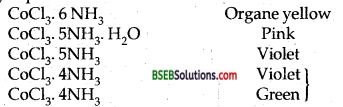Different colours of CoCl3.4NH3 compound is due to the fact that they are cis and trans isomers.Question 2.
FeSO4 solution mixed with (NH4)2 SO4 solution in 1:1 molar ratio gives the test of Fe2+ ion but CuSO4 solution mixed with aqueous ammonia in 1:4 molar ratio does not give the test of Cu2+ ion. Explain why?
FeSO4 solution mixed with (NH4)2 SO4 solution in 1:1 molar ratio gives rise to FeSO4. (NH4)2SO4. 6H2O
which is a double salt
FeSO4(aq)+(NH4)2SO4(aq)+6H2O → FeSO4.(NH4)2SO4.6H2O (Mohr’s salt)
which loses its identity in aqueous solution giving individual ions Fe2+, NH4 and SO42- ions. Therefore Fe2+ion gives its test. On the other hand CuSO4 solution mixed with NH3 in 1:4 molar ratio gives rise to the coordination compound [CU(NH3)4]SO4 which being a complex compound does not gives the test of Cu2+ ions.
CuSO4 + 4 NH3 → [Cu (NH3)4]SO4 [CU (NH3)4]2+
retains its identity in aqueous solution.

Question 3.
Explain with two examples each of the following: Coordination entity, ligand, coordination number, coordination 1 polyhedron, homoleptic and heteroleptic.
Coordination Entity or a complexion may be defined an as electrically charged or neutral species which consists of a central metal atom or ion surrounded by a group of ions or neutral molecules. For example [Ni (NH3)6]2+ is a complexion or coordination entity in which the central Nickel ion Ni2+ is surrounded by six NH3 molecules. Similarly [Ag(CN)2] in which central ion Ag+ is surrounded by two CN ions. It may also be a neutral molecule like [Co (NH3)3Cl3], [Ni (CO)4].

Ligand-The neutral molecules or anions attached to the central metal atom or ion is called the Ligand. In the coordination entity [ Ag(NH3)2]+, the central atom is Ag (I) ion and two ammonia molecules are Ligands.
[CO(CN)6]3- CO3+: central metal ion There are 6 CN ions which act as ligands.
To be a ligand, it must have at least one atom which is capable of donating a pair of electrons to the central metal atom/ion. For example
N in: NH3 and: C≡ N – in above examples.

Coordination Number-The coordination number of; a metal ion in a complex can be defined as the number of ligand donor atoms to which the metal atom/ion is directly attached. For example, in the complexions [Pt Cl6]2- and [Ni (NH3)4]2+, the coordination number of Pt and Ni are 6 and 4 respectively. Similarly, in the complexions, [Fe (C2O4)3]2- and [Co (en)3]3+ the coordination number of Fe and Co both is 6 because C2 O42- and en (ethane-1,2-diamine) are dentate ligands.

Coordination Polyhedron-The spatial arrangement of the ligand atoms which are directly bonded to the central atom/ion defines coordination polyhedron about the central atom /ion. The most common coordination polyhedra are octahedral in [Co (NH3)6]3+; tetrahedral in [Ni (CO)4] and square planar in [PtCl4]2-.Homoleptic and Heteroleptic Complexes-Complexes in which a metal is bound to only one kind of donor groups, e.g., [Co(NH3)6]3+ are known as homoleptic. Complexes in which a metal is bound to more than one kind of donor groups, e.g., [Co(NH3)4Cl2] are known as heteroleptic.Question 4.
What is meant by unidentate, bidentate and ambidentate ligands? Give two examples for each.
Unidentate Ligand is one which has only one donor atom present in it which can coordinate with the metal atom/ion. NH3 and H2O are the examples of unidentate ligands as they have only one kind of donor atoms in cm: Nitrogen in NH3 and Oxygen in H2O.
Bidentate ligand—If a ligand has two donor atoms in it which it can coordinate with the metal, it is called a bidentate ligand. For example ethylenediamine (en) and oxalate in (ox).Ambidentate ligandUnidentate ligands containing more than one coordinating atoms are called ambidentate ligands. For example
NO2 can coordinate through either nitrogen or oxygen. Similarly CN can coordinate through either carbon or nitrogen.

Question 5.
Specify the oxidation numbers of the metals in the following coordination entities:
(a) [Co (H2O(CN) (en)2]2+,
(b) [Pt Cl4]2-
(c) [Cr (NH3), Cl3],
(d) [Co Br2 (en)2]2+
(e) K3[Fe (CN)6
(a) [Co (H2O(CN) (en)2]2+In the complex H2O and (en) are neutral ligands. Let the ox. no. of Co be x.
xt(-1) = +2
x = + 3
ox. no. of Co = + 3

(b) [Pt Cl4]2- Let the ox. no. of Pt = x.
∴ x + 4 (- 1) = -2
x = 4-2 =+2
∴ ox. no. of Pt = +2

(c) [Cr (NH3), Cl3]-Let the ox. no. of Cr be x. Here NH3 is a neutral ligand.
∴ x + (- 3) = 0 ‘
x = + 3
∴ ox. no. of Cr = +3

(d) [Co Br2 (en)2]2+-Let ox. no. of Co = x. Here (en) is a neutral ligand.
∴ x + 2 (-1) = +1
∴ x = + 3
∴ ox.no. of Co = +3

(e) K3[Fe (CN)6-Here ox. no. of K = + 1 and CNT = -1.
Let ox. no. of Fe be x
∴ +3(+1) + x + 6(-1) =0
+3+x-6 = 0
or x = + 3
ox. no. of Fe = +3.

Question 6.
Using IUPAC norms write the formulae for the following :
(i) Tetrahydroxozincate (III)
Tetrahydroxozincate (II) [Zn (OH)4]2-
(iii) Diamminedichlorodoplatinum (II)
Diamminedichlorodoplatinum (II) [PtCl2 (NH3)2]
(iv) Potassium tetra cyanonickelate (II)
Potassium tetra cyanonickelate (II) K2[Ni(CN)4]
(v) Pentaamminenitrito-O-cobalt (III)
Pentaamminenitrito-O-cobalt (III) [Co (ONO) (NH3)5]2+.
(vi) Hexaamine cobalt (III) sulphate
Hexamine cobalt (III) sulphate [Co(NH3)6]2(SO4)3.
(vii) Potassium tri (oxalato) chromate (III)(viii) Hexaamineplatinum (IV)
Hexaamineplatinum (IV). [Pt (NH3)6]4+
(ix) Tetrabromodocuprate (II)
Tetrabromodocuprate (II) [Cu Br4]2-
(x) Penetaamminenitrito-N-cobalt (III).
Pentaamminenitritro-N-cobalt (III) [Co (NO2) (NH3)5]2+.

Question 7.
Using IUPAC norms write the systematic names of the following:
(i) [Co (NH3)6]Cl3,
(ii) [Pt (NH3)2Cl(NH2CH3)]Cl,
(iii) [Ti(H2O)6]3+,
(iv) [Co (NH3)4 Cl (NO2)] Cl,
(V) [Mn (H2O)6]2+,
(vi) [NiCl4]2-
(vii) [Ni (NH3)6]Cl2,
(viii) [Co (en)3]3+,
(ix) [Ni (CO)4].
(i) [Co (NH3)6]Cl3: Hexamminecobalt (III) chloride.
(ii) [Pt (NH3)2Cl(NH2CH3)]Cl : Diammine amminomethane chloroplatinum (II) chloride.
(iii) [Ti(H2O)6]3+: Hexaaquatitamium (III) ion
(iv) [Co (NH3)4 Cl (NO2)] Cl : Tetraamminechloronitro-N-cobalt (III) chloride.
(v) [Mn (H2O)6]2+: Hexaaquamanganese (II) ion.
(vi) [NiCl4]2-;Tetrachlomickelate(II)
(vii) [Ni (NH3)6]Cl2: Hexaamine nickel (II) chloride
(viii) [Co (en)3]3+: Tris (ethane-1,2-diammine) cobalt (III) ion.
(ix) [Ni (CO)4]: Tetracarbonyl nickel (O).Question 8.
List various types of isomerism possible for coordination compounds, giving an example of each.
There are two types of isomerism possible for coordination compounds.
A. Stereoisomerism arises when two or more compounds have the same chemical formula and chemical bonds but have different spatial arrangements of atoms. They are further of two types:
(i) Geometrical isomerism,
(ii) Optical isomerism.
(i) Geometrical Isomerism-It arises in heteroleptic complexes due to different possible geometrical arrangements of ligands for example the square planar coordination compound [Pt (NH3)2. Cl2] exists in two forms-cis and trans.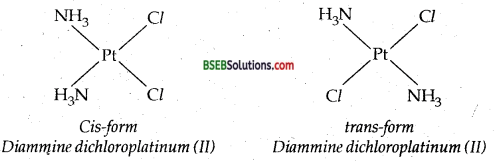(ii) Optical isomerism arises in dissymmetrical (which lack symmetry) molecules. The optical isomers rotate the plane of polarised light equally but in opposite direction. Those which rotate the plane polarised light towards left are called laevorotatory or 1- or (-) form. Those which rotate the plane polarised light towards right are called dextro-rotary or d- or (+) form. An example is given by coordinating entity [CrCl2(en) (NH3)2]+.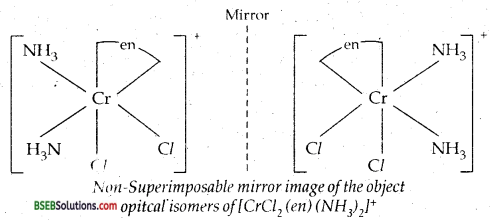Here en represents H2N-CH2-CH2-NH2 One of the enantiomers is dextro and the other is laevo.

B. Structural isomerism-It is further divided into the following: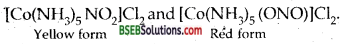(ii) Coordination isomerism-
[Co (NH3)6] [Cr(CN)6] and [Cr (NH3)6] [Co (CN)6]

(iii) Ionisation Isomerism-(iv) Hydrate or Solvate Isomerism-[Cr(H2O)6]Cl3 is violet and [Cr(H2O)5Cl]Cl2. H2O is grey-green.

Question 9.
How many geometrical isomers are possible in the following coordination entities?
(a) [Cr(C2O4)3]3-,
(b) [CO(NH3)3 Cl3]
(a) No geometrical isomers are possible for the coordination entity [Cr (C2O4)3]3-.
(b) There are two geometrical isomers possible for [CO(NH3)3 Cl3]: fac and mer.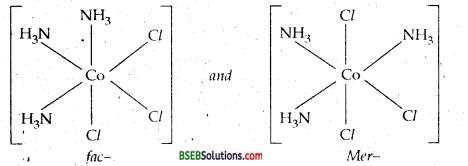Question 10.
Draw the structures of optical isomers of
(a) [Cr (C2O4)3]3-,
(b) [Pt Cl2 (en)2]2+,
(c) [Cr(NH3)2 Cl2 (en)]+.
(a) The two optical isomers of [Cr (C2O4)3]3- are C2O42- short ox.(b) The two optical isomers of [Pt Cl2(en)2]2+ are:(c) [Cr (NH3)2 Cl2 (en)]+: The two optical isomers are: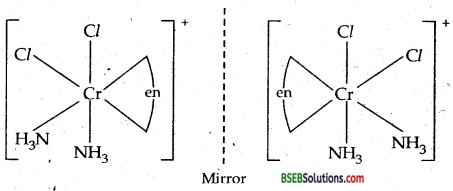Question 11.
Draw all the isomers (geometrical and optical) of:
(i) [CoCl2(en)2]+
(ii) [Co(NH3)Cl(en)2]2+
(iii) [Co(NH3)2Cl2(en)]+
(i) [CoCl2(en)2]+Geometrical isomers (Cis and trans) of [CoCl2(en)2]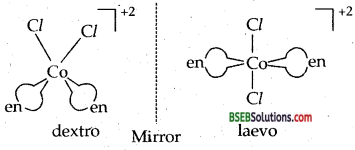(ii) [Co(NH3)Cl(en)2]2+optical isomers d and l of cis [Co(NH3)Cl(en)2](iii) [Co(NH3)2Cl2(en)]+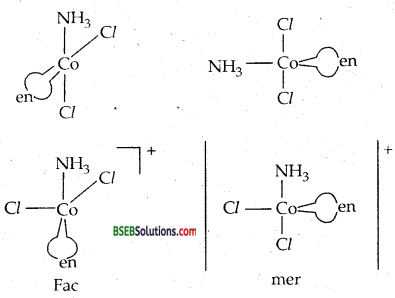Question 12.
Write all the geometrical isomers of [Pt (NH3) Br Cl (Py)] and how many of them will exihibit optical isomers?
Various geometrical isomers of [Pt (NH3) Cl Br (Py) are three :Two are cis and one is trans.Question 13.
Aqueous copper sulphate solution (blue in colour) gives (i) a green precipitate with aqueous potassium fluoride and (ii) a bright green solution with aqueous potassium chloride. Explain these experimental results.
Aqueous CuSO4 solution exists as [Cu (H2O)4]SO4 which has blue colour due to [Cu (H2O)4]2+ ions.
(i) When KF is added, the weak H2O ligands are replaced by F ligands forming [CuF4]2- ions which is a green precipitate.
[Cu (H2O)4]2+ + 4F → [CuF4]2- + 4 H2O
(ii) When KCl is added, Cl ligands replace the weak H2O ligands forming [Cu Cl4]2- ions which has bright green colour.
[Cu (H2O)4]2+ + 4 Cl → [CuCl4]2- + 4 H2O.

Question 14.
What is the coordination entity formed when excess of aqueous KCN is added to an aqueous solution of copper sulphate? Why is it that no precipitate of copper sulphide is obtained when H2S (g) is passed through this solution?
When KCN (aq) or CN ions are added in excess to an aq. CuSO4 solution, the complex entity [Cu(CN)4]2- is formed.
[Cu (H2O)4]2+ + 4 CN → [Cu (CN)4]2- + 4 H2O
As CN is a strong ligand, it forms a highly stable complex with Cu2+ ion. On passing H2S, free Cu2+ ions are not available to form the precipitate of CuS.

Question 15.
Discuss the nature of bonding in the following coordination entities on the basis of valence bond theory.
(a) [Fe(CN)6]4-
(b) [FeF6]3-
(c) [Co (C2O4)3]3-
(d) [CoF6]3-.
(a) [Fe(CN)6]4-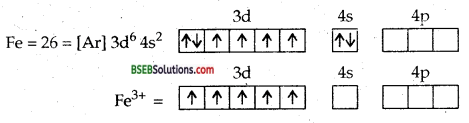CN is a powerful ligand, it pushes the 5d electrons in three 3d orbitals forcing them a pair up leaving one electron unpaired leaving two out of five 3d orbitals free to take part in d2sp3 hybridization.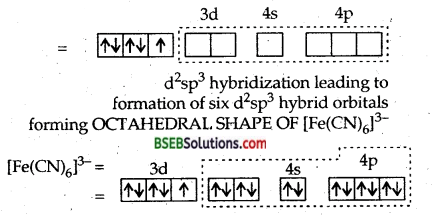Six CN ligands deposit six pair of electrons in six d2sp3 hybrid orbitals. The molecule is LOW SPIN and PARAMAGNETIC.
(b) [FeF6]3- Hexafluoro ferrate (III)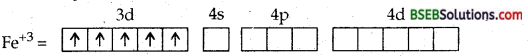F is a weak ligand (or ligand of weak field strength). It is not in a position to push the two electrons in the last two d orbitals backwards and force them to pair up.
∴Inner two d orbitals cannot be used for hybridization. Thus, here 4d orbitals will be used for hybridization, viz., sp3d2 resulting in the formation of octahedral complex. This complex will be called high spin complex or OUTER ORBITAL COMPLEX.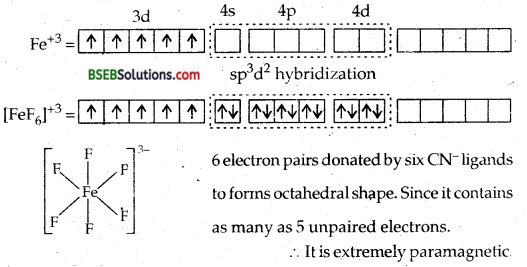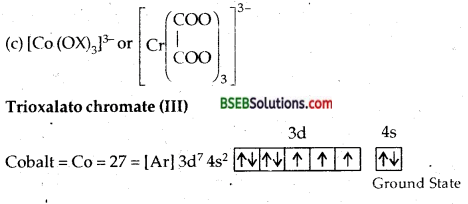the 4 electrons in four 3d orbitals into two 3d orbitals rendering two 3d oroitals vacant and thus free to take part in d2sp3 hybridization. It will lead to Octahedral complex. Since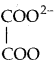is a bidentate ligand, therefore 6 electron pairs from three ligands will be accomodated in six d2sp3 hvbrid orbitals. Since the complex does not contain any unpaired electrons.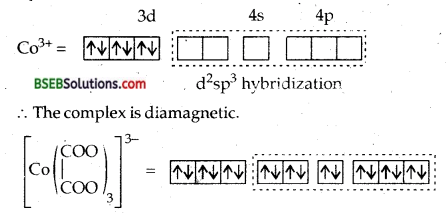It is outer orbital complex and is octahedral as shown below :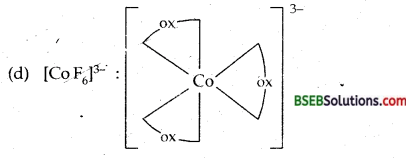(d) [Co F6]3--Hexafluorocobaltate (III) ion-In this complex the outer electronic configuration of cobalt in excited state i.e., under conditions of formation of complex is 3d6. Since F ion provides a weak ligand field therefore, one 4s, three 4p and two 4d orbitals hybridise to yield six sp3 d2 hybrid orbitals pointing towards the six ends of an octahedron. The six F ions then donate a pair of electrons to each of these vacant orbitals to have an octahedral geometry. The presence of unpaired electrons in 3d orbitals makes the complex paramagnetic. The schematic formation of complex is illustrated below:Since the formation of the complex involves d-orbitals of the valence or outer shell hence it is called OUTER ORBITAL OR HIGH SPIN OR HYPOLIG ATED COMPLEX.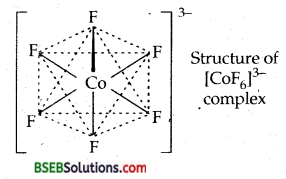Question 16.
Draw figure to show the splitting of d-orbitals in an octahedral crystal field. ,
Fig. below shows the splitting of degenerated-orbitals in an octahedral complex on the approach of ligands towards the metal atom/ion.Question 17.
What is spectrochemical series? Explain the difference between a weak field ligand and a strong field ligand.
The crystal field splitting (A) energy depends upon the field strength of the ligands which is in the order:
I < Br < SCN < Cl < S2- < F < OH < C2O42- < H2O < NCS <
eda4- < NH3 < en < CN < CO.
Such a series is called Spectrochemical series.
I is the weakest ligand and CO is the ligand with the maximum field strength.
(i) In the case of ligands of weak field like I.
Δ < P where P is called pairing energy. It is the energy required to pair up the electrons in a single orbital. The complex formed is usually high spin.
(ii) In the case of strong field ligand like CN or CO, Δ > P. The complex formed is low spin.Question 18.
What is crystal field splitting energy? How does the magnitude of A0 decide the actual configuration of d-orbitals in a coordination entity?
The splitting of five d-orbitals of metal atom/ion on the approach of the ligand^ towards the metal atom/ion for the purpose of bond formation is called crystal field splitting. Those d-orbitals of central atom/ion which lie on the direct path of die ligands (e.g. set in octahedral complex) are repelled more than those which lie away in between the axes x, y, z (e.g.t2g set of dxy/ dyz, dzx orbitals in an octahedral complex).

The difference of energy between the two sets e and t2g is called crystal field splitting energy. If the value of Δ0 is high, complex formed is low spin as the inner two d-orbitals hybridize with s and three p orbitals to give d2sp3 hybridization and the complex formed.is inner-orbital complex. If the value of Δ0 is low the two d-orbitals of nth shell mix up with nth and three np orbitals to give sp3d2 hybridization and the complex formed is called outer-orbital complex.

Question 19.
[Cr(NH3)6]3+ is paramagnetic while [Ni (CN)4]2- is diamagnetic. Explain why?
Ans. ft can be explained on the basis of valence bond theory that [Cr(NH3)6]3+ is paramagnetic whereas [Ni (CN)4]2- is diamagnetic.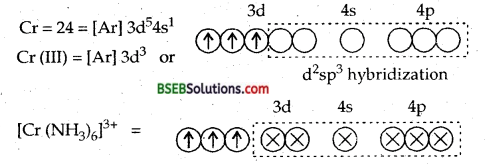6NH3 ligands deposits their six pairs of electrons in dsp3 hybrid orbitals. Since there are three unpaired electrons in the 3d-orbitals, therefore [Cr(NH3)6]3+ is paramagnetic.∴ It will push two impaired electrons backwards in the same orbital rendering one 3d orbitals vacant which can undergo hybridization with one 4s and two 4p orbitals giving 4 dsp2 hybrid orbitals.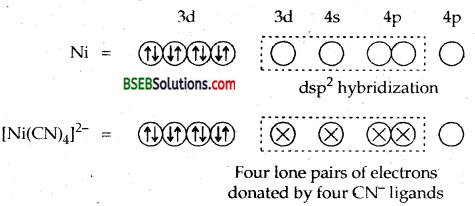Since the complex does not contain any unpaired electrons it is diamagnetic.

Question 20.
A solution of [Ni (H2O)6]2+ is green but a solution of [Ni (CN)4] is colourless. Explain.
Ni2+ ion in [Ni (H2O)6]2+ is green in colour because it has two unpaired electrons in the 3d-orbitals and due to d-d transition complex is green in colour. On the other hand [Ni (CN)4]2- has no unpaired electrons in the 3d-orbitals [3d10 configuration], hence it is colourless.

Question 21.
[Fe (CN)6]4- and [Fe(H2O)6]2+ are of different colours in dilute solutions. Why?
[Fe (CN)6]4- has no unpaired electrons due to d2sp3 hybridisation. All electrons in 3d orbitals are paired, whereas in [Fe(H2O)6]2+, there are four unpaired electrons in 3d orbitals and the hybridization involved in sp3d2. In this complex d-d transitions are possible and therefore, it shows different colour.

Question 22.
Discuss the nature of bonding in metal carbonyls.
Bonding in metal Carbonyls-The homoleptic carbonyls are formed by most of the transition metals like Ni, Fe, Cr, Mn, Co as shown below: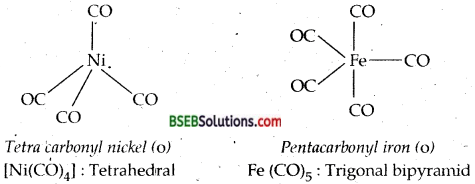Decacarbonyldimanganese (O) is made up of two square pyramidal Mn (CO)5 units joined by a Mn-Mnbond. Octacarbonyldicobalt (O) has a CO-CO bond bridged by two CO groups.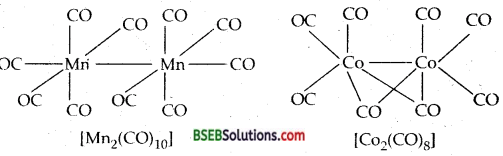The metal-carbon bond in metal carbonyls possess both σ and π character. The M-C σ bond is formed by the donation of lone pair of electrons on the carbonyl carbon into a vacant orbital of the metal atom. The M-C π bond is formed by the donation of a pair of electrons from a filled d orbital into the vacant antibonding π orbital of CO. The metal to ligand bonding creates a synergic effect which strengthens the bond between CO and the metal.Question 23.
Give the oxidation state, d orbital occupation and coordination number of the central metal ion in the following complexes:
(i) K3[Co(C2O4)3]
(ii) (NH4)2 [Co F4]
(iii) Cis-[Cr (en)2 Cl2)]Cl
(iv) [Mn (H2O)6]SO4.
(i) Oxidatin state of Co = +3; C.Ñ. =6, d-orbitaI occupation is t62ge0g.
(ii)O.S. of Co = + 2, C.N. = 4; d7occupancy is t52ge2g
(iii) US. of Cr = + 3, CN. = 6; d,3 occupacy is t32g
(iv) O.S. of Mn = + 2 C.N. = 6; d5 occupacy is t32ge2g.

Question 24.
Write down the IUPAC name for each of the following complexes and indicate the oxidation state, electronic configuration and coordination number. Also give stereochemistry and magnetic moment of the complex.
(i) K[Cr (H2O)2 (C2O4)2]. 3H2O,
(ii) CrCl3 (py)3,
(iii) K4 [Mn (CN)6],
(iv) [Co (NH3)5 Cl] Cl2,
(v) Cs [Fe Cl4].
(i) Potassium diaquadioxalatochromate (III) trihydrate. C.N. = 6
Ox. State = + 3; electronic configuration of Cr3+ = 3d3 [ t32g e2 ]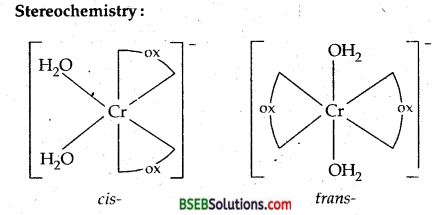Since it contains three unpaired electrons 3d3.
µmag= $$\sqrt{n(n+2)}$$ = $$\sqrt{3(3+2)}$$
= $$\sqrt{15}$$ =3.87 B.M.

(ii) [Cr Cl3 (Py)3] I.U.P.A.C. name Trichlorotripyridine chromium (III).
O.S. of Cr = + 3
C.N. = 6 Electronic config. of cr3+ = 3d3 [ t32g e2 ].
Stereochemistry:
(i) Geometrical isomers-(ii) Another type of geometrical isomerism is facial (fac) and meriditional (mer) isomers.
Magnetic moment (µ): It contains 3 impaired electrons.
µ = $$\sqrt{n(n+2)}$$ = $$\sqrt{(3+2) \times 3}$$ = $$\sqrt{15}$$ =3.87BM.

(iii) K4 [Mn(CN)6] : I.U.P.A.C. name is Potcssiumhexa- cyanomanganate (II) O. state of Mn = + 2, C.N. = 6
Electronic config. of Mn = 3d5 [t32g e2 ]
Octahedral complex: No Stereoisomerism: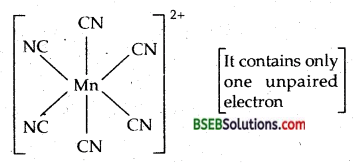Magnetic moment-µ = $$\sqrt{n(n+2)}$$ = $$\sqrt{1(1+2)}$$ = 1.73 BM.
(iv) [CO(NH3)5 Cl]Cl2-IUPAC name is Pentaamminechlorocobalt (III) chloride. Ox. state of Co = + 3; C.N. = 6. El. conf. = 3d6 [t52g e0g ].
Octahedral complex-Magnetic moment = 0, as there are no unpaired electrons.

(v) Cs[FeCl4]-I.U.P.A.C. name is Cesium tetrachloroferrate (III).
Ox. State of Fe = + 3; C.No. = 4; El. conf. = 3d5 (t32g e2)
It is a tetrahedral complex.µ= $$\sqrt{n(n+2)}$$ =$$\sqrt{5(5+2)}$$ = $$\sqrt{35}$$ = 5.9 BM
[as it contains 5 unpaired electrons.]

Question 25.
What is meant by stability of a coordination compound in solution? State the factors which govern stability of complexes.
The stability of a complex in solution refers to the degree of association between the two species involved in the state of equilibrium. The magnitude of the (stability or formation) equilibrium constant for the association; quantitatively expresses the stability. Thus, if we have a reaction of the type.
M + 4 L → ML4
K = $$\frac{\left[\mathrm{ML}_{4}\right]}{[\mathrm{M}][\mathrm{L}]^{4}}$$
larger the stability constant, the higher the proportion of ML4 that exists in solution.

Factors on which stability of the complex depends-

• Charge on the central metal ion-Greater the charge on the central metal ion, greater is the stability of the complex.
• Nature of the metal ion-Groups 3 to 6 and inner transition elements form stable complexes when donor atoms of the ligands are N, O and F. The elements after group 6 of the transition metals which have relatively d-orbitals (e.g, Rh, Pd, Ag, Au, Hg etc.) form stable complexes when the donor atoms of the ligands are heavier members of N, O and F family.
• Basic nature of the ligand-Greater the basic strength of the ligand, greater is the stability of the complex.
• Presence of chelate rings in the complex increases its stability. It is called chelate effect. It is maximum for the 5- and 6-membered rings.
• Effect of multidentate cyclic ligands—If the ligands happen to be multidentate and cyclic without any steric effect, the stability of the complex is further increased.

Question 26.
What is meant by the Chelate effect? Give an example.
Presence of chelate rings in the complex increases the stability of the complex. It is called chelate effect.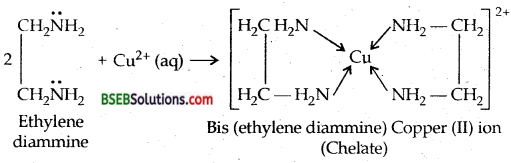Question 27.
Discuss briefly giving an example in each case the role of coordination compounds in
(i) biological systems,
(ii) analytical chemistry,
(iii) medicinal chemistry and
(iv) extraction/metallurgy of metals.
(i) Biological Systems-The pigment (green) chlorophyll is responsible for photosynthesis. It is a coordination compound of magnesium. Haemoglobin, the red pigment of blood which acts as oxygen carrier is a coordination compound of iron: Vitamin B12, Cyana cobalt mine, the anti-pernicious anaemia factor is a coordination in compound of cobalt. Other compounds of biological importance are enzymes.

(ii) Analytical Chemistry-Coordination compounds find use in many qualitative and quantitative chemical analysis. The familiar colour reactions given by metal ions with a number of -ligands (especially chelating ligands), as a result of formation of coordination entities, form the basis for their detection and estimation by classical and instrumental methods of analysis. Examples of such reagents include EDTA, DMG (dimethylglyoxime), a-nitroso-P-naphthol, coupon etc.

(iii) Medicinal Chemistry-There is growing interest in the use of chelate therapy in medicinal chemistry. An example is the treatment of problems caused by the presence of metals in toxic proportions in plant/ animal systems. Thus, excess of copper and iron are removed by the chelating ligands D-penicillamine and desferrioxamine B via the formation of coordination compounds. EDTA is used in the treatment of lead poisoning. Some coordination compounds of platinum effectively inhibit the growth of tumours. Examples are: ris-platin and related compounds.

(iv) Extraction/metallurgy of metals-Some important extraction processes of metals, like those of silver and gold, make use of complex formation. Gold, for example, combines with cyanide in the presence of oxygen and water to form the coordination entity [Au(CN)2] in aqueous solution. Gold can be separated in metallic form from this solution by the addition of zinc.

Question 28.
How many ions are produced from the complex Co (NH3)6 Cl2 in solution? ^
(i) 6,
(ii) 4,
(iii) 3,
(iv) 2.
(iii) 3.

Question 29.
Amongst the following ions which one has the highest magnetic moment value?
(i) (Cr (H2O)6]3+,
(ii) [Fe(H2O)6]2+
(iii) [Zn((H2O)6]2+
(ii) As [Fe (H2O)6]2+ has maximum no. (4) impaired electrons out of them.

Question 30.
The oxidation number of cobalt in K [Co (CO)4] is (i) + 1,(ii) + 3,(iii)-l,(iv)-3.
(iii)-1.

Question 31.
Amongst the following the most stable complex is (i) [Fe (H2O)6]3+,
(ii) [Fe(NH3)6]3+,
(iii) [Fe (C2O4)3-
(iv) [Fe Cl6]3-.
(iii) [Fe (C2O4)3-.Question 32.
What will be the correct order for the wavelengths of absorption in the visible region for the following: (Ni (NO2)6]4-, [Ni (NH3)6]2+, [Ni (H2O)6]2+.
The order of the ligands in the spectrochemical series: H2O < NH3 < NO2. Hence the wavelength of the light absorbed will be in order:
[Ni (H2O)6]2+< [Ni (NH3)6]2+ < [Ni (NO2)6]4-.
Thus the wavelengths absorbed [E = $$\frac{\mathrm{hc}}{\lambda}$$] will be in the opposite order.

### Bihar Board Class 12 Chemistry Coordination Compounds Additional Important Questions and Answers

Question 1.
Which isomer of [CoCl2 (en)2]+ does not show optical isomerism?
Trans isomer does not show optical isomerism.

Question 2.
Out of tetra carbonyl nickel (O) and tetramine copper (II) sulphate which is paramagnetic and why? (P.S.B. 2005)
Tetraamine copper (II) sulphate is paramagnetic because it has one unpaired 3d electron, whereas tetracarbonyl nickel (O) is diamagnetic as it has all paired electrons.

Question 3.
Why only transition metals are known to form Jt complexes? (D.S.B. 2002)
Transition metal .ions have empty d-orbitals into which electron pairs can be donated by ligands containing n electrons, e.g., CH2 = CH2 = CH2,C5H5, C6H6 etc.

Question 4.
Do we call metal carbonyls as organometallics? Why or Why not?
Yes, metal carbonyls are called organometallics because C atom of CO is linked to the metal atom. If fact, the metal-carbon bonds have both a and n characters.

Question 5.
The values of dissociation constants of [Cu (NH3)4]2+ and [Co (NH3)6]3+ are 1.0 × 10-12 and 6.2 × 10-36 respectively. Which complex will be more stable and why? (D.S.B. 2004 C)
Smaller the value of dissociation constant, more stable is the complex in solution. Hence [Co (NH3)6]3+ is more stable than [Cu (NH3)4]2+.

Question 6.
Write the structure of ferrocene. [H.S.B. 97,2000,2001]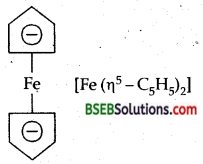Question 7.
In the formula [Fe (η5 – C5H5)2] what does the prefix η5 denote? (A.I.S.B. 99)
η5 denotes that all the five carbon atoms of C5H5 are linked to the central metal atom Fe.Question 8.
Name the IUPAC names of the following.
(i) [Pt(NH3)2Cl2]Cl2
(ii)[Cr(NH3)4Cl2]+ (D.S.B.2001)
(i) Diamminedichloroplatinum (IV) chloride.
(ii) Tetraammine di chlorochromium (III) ion.

Question 9.
Name the ionisation isomer of (Cr (H2O)5 Br)SO4 (A.I.S.B. 2002)
Pentaaquasulphatochromium (III) bromide (Cr (H2O)5 Br)SO4.

Question 10.
What is the oxidation state of Ni in [Ni (CO)4]? (AISB 95, HSB 01)
Oxidation state of Ni is 0.

Question 11.
Which type of ligands form chelates?
Polydentate ligands.

Question 12.
Give an example of Chelate complex. (H.S.B. 2001)
Bis (ethylenediamine) copper (II) sulphate.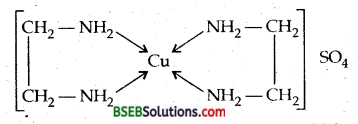Question 13.
Give two names of two complexes which are used in medicines. (D.S.B. 2005 C)

• EDTA, used in the treatment of lead poisoning.
• Cis-platin [Pt Cl2 (NH3)2], used in the treatment of cancer.

Question 14.
Give the geometry and magnetic character of [Ni Cl4]2-
Tetrahedral, Paramagnetic.

Question 15.
What are ambidentate ligands. Give an example. (AISB 2005 C, HSB 2005)
Unidentate ligands containing more than one coordinating atoms are called ambidentate ligands. For example, NO2- can coordinate through nitrogen or oxygen.

Question 16.
Why complexes are preferred in the electrolytic bath for electroplating?
They dissociate slowly and hence give a smooth and even deposit.

Question 17.
What is a bidentate ligand?
A ligand which contains two donor atoms so that it can form two coordinate bonds with the central metal atom, e.g., ethylene, diammineNH2-CH2-CH2-NH2.

Question 18.
What is the coordination number of Fe in [Fe (EDTA)]?
Six.

Question 19.
What is the coordination number of central metal ion in [Fe(C2O4)3]3-?
Six.Question 20.
What is crystal field splitting energy?
The difference of energy between the two sets of d-orbitals after splitting on the approach of the ligands is called crystal field splitting energy.

Question 1.
The species [CuCl4]2- exists, but [Cul4]2- does not. Explain why? (I.I.T.92)
This is because atom is very large as compared to Cl in size. Copper is not in a position to accommodate four such big atoms around it.

Question 2.
Write the formulae for the following coordination compounds:
(i) tetra hydroxozincate (II),
(ii) tetrabromocuprate (II),
(iii) Potassium tetracyanocuprate (II),
(iv) Pentacarbonyl iron (O).
(i) [Zn (OH)4]2-,
(ii) [CuBr4]2-,
(iii) K2 [Cu (CN)4]
(iv) [Fe (CO)5].

Question 3.
Which out of the following two coordination entities is chiral optically active)?
(a) cis – [CrCl2 (OX)2]
(b) trans- Cr[Cl2 (OX)2]3-
The two entities are represented as: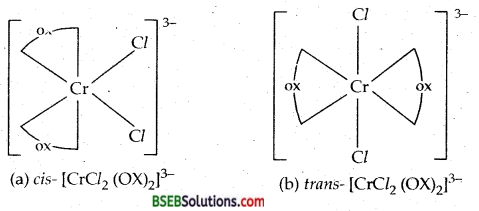Out of the two, (a) cis – [CrCl2 (OX)2]3- is chiral (optically active).

Question 4.
Predict the number of unpaired electrons in the square planar [Pt (CN)4]2- ions.
Pt2+ is a d8 ion. For square planar geometry dsp2 hybrids are required. For the availability of are d orbital, pairing of electrons takes place in the remaining d-orbitals. Hence there are no unpaired electrons in [Pt(CN)4]2- ion.

Question 5.
Which of the two compounds is more stable and why?
K4[Fe(CN)6],K3[Fe(CN)6].
K3[Fe(CN)6] is more stable than K4[Fe(CN)6], because in the former entity Fe is in oxidation state III while in the latter, it is an oxidation state II, which can be oxidized to more stable oxidation state III.

Question 6.
Why [Ti (H2O)6]3+ is violet in colour.
Ti3+ has one electron in 3d orbital.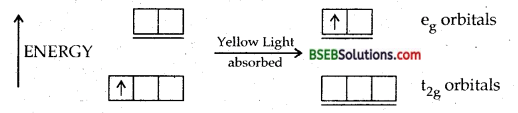Yellow portion of light is absorbed.
The solution of [Ti (H2O)6]3+ is violet.

Question 7.
[Co (CN)6]3- and [Co F6]3+ both are octahedral complexes. Then what is the difference between them?
[Co (CN)6]3- is an inner-orbital complex or low spin complex involving the d2sp3 hybridization while [Co F6]3+ is an outer orbital or high spin complex involving sp3d2 hybridization.

Question 8.
Explain how [Pt (NH3)2Cl2] and [Pt (NH3)6]Cl4 differ in their electrolytical conductances?
[Pt (NH3)2Cl2] is a non-conductor as it furnishes nil ions in the solution while [Pt (NH3)6]Cl4 conducts and its molar conductance is expect to correspond to a total number of 5 ions [1:4 electrolyte].

Question 9.
[Pt(NH3)6]Cl4 ⇌ [Pt (NH3)6]4+ + 4Cl
The complex CoBr3. 4NH3. 2H2O has molar conductivity corresponding to 3:1 electrolyte. Write the molecular formula and its I.U.P.A.C. name.
Since the molar conductivity corresponds to 3:1 so the formula is [Co (NH3)4 (H2O)2] Br3 ⇌ [Co (NH3)4 (H2O)2]3+ + 3Br bromide.Question 10.
Giving examples, give the two categories of organometallics. .
Organometallics compounds are of two types a and n bonded.

1. a bonded organometallics: R-Mg-XR = alkyl radical,
X = Halogen; Diethyl Zinc (C2H5)2 Zn, Al (CH3)3.
2. π bonded organometallics: Zeise salt K [PtCl3 (C2H4)] :
K [PtCl3 η2 – C2H4], ferrocene [Fe η5 – C5H5)2].

Question 11.
Which is more paramagnetic and why? [Fe (H2O)6]3+ [Fe(CN)6]3-.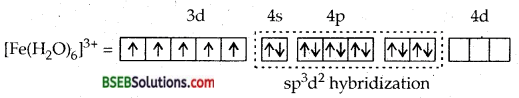It is outer orbital complex.
HIGH SPIN. There are 5 unpaired electrons, H2O being a weak ligand. Can’t pair up the electrons.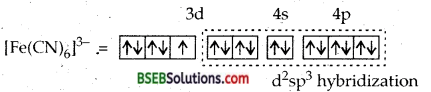It is inner orbital or LOW SPIN COMPLEX. CN being a powerful ligand pushes the 5d electrons in three d orbitals leaving two d orbitals free for hybridization. Only one unpaired electron makes it less paramagnetic.

Question 12.
How will you prepare [Ni (CO)4]? What is the oxidation number of nickel in it? Give its main use.
Ni + 4 CO → [Ni (CO)4]
Ox. No. of Ni = 0
It is used for the purification of Nickel by MOND’s process.

Question 13.
Using valence bond theory explain the geometry and magnetic behaviour of pentacarbonyl iron (O). (D.S.B. 2005 C)Hence [Fe (CO)5] has trigonal bipyramidal geometry. Since it does not have any unpaired electrons, it is diamagnetic.

Question 14.
A coordinate compound has the formula CoCl3.4NH3. It does not liberate ammonia but precipitates chloride ions as silver chloride. Give IUPAC name of the complex and write its structural formula. (A.I.S.B. 1998,2004 C)
Remembering that the coordination number of cobalt is six, the formula of the complex will be [CoCl2 (NH3)] Cl.
Its IUPAC name will be tetrammine dichlorocobalt (III) chloride.

Question 15.
Square planar complexes with coordination number of four exhibit geometrical isomerism whereas tetrahedral complexes do not. Why?
Tetrahedral complexes do not show geometrical isomerism because the relative positions of the ligands attached to the central metal atom/ion are same with respect to each other.

Question 16.
Give a chemical test to distinguish between [Co (NH3)5 Br]SO4 and [Co (NH3)5 SO4] Br. Name the type of isomerism exihibited by them.
The complex [Co (NH3)5 Br]SO4 will give SO42- ions in solution which give white ppt. on reaction with BaCl2 solution. The second complex will give Br ions in the solution which give yellowish-white precipitate with AgNO3 solution. They exihibit ionisation isomerism.

Question 17.
What type of isomerism is shown by the following complexes? (H.S.B. 2004)
(i) [Cr(en)3]3+,
(ii) [Pt (NH3)4] [Pt Cl4]
(i) [Cr(en)3]3+ shows optical isomerism.
(ii) Coordination isomerism, because some Cl and NH3 ligands may interchange, e.g., [Pt Cl(NH3)3] [Pt Cl3 (NH3)].

Question 1.
Discuss the main postulates of valence bond theory as applicable to coordination compounds, giving examples of octahedral and tetrahedral coordination compounds.
Assumption:
1. The ligand must have a lone pair of electrons to donate to the central metal atom/ion.
2. The metal atom ion must have empty sites to accept lone pair of electrons donated by LIGANDS.
Valence Bond Theory-The valence bond treatment of coordination compound was developed by Pauling, The brief outlines of the theory are as follow:

(i) A suitable number of vacant orbitals must be present on the central metal atom for the formation of coordinate bonds with suitable ligand orbitals.
(ii) Depending on the total number of bonds to be formed the central metal ion can use appropriate number of atomic orbitals i.e., s, p or d for hybridisation yielding a set of equivalent orbitals called hybrid orbitals.
(iii) The hybridised orbitals are then allowed to overlap with those ligand orbitals that can donate an electron pair for bonding.

(iv) The outer orbital (high spin) or inner orbital (low spin) complexes are formed depending upon whether the d-orbital of outer shell or the d-orbitals of the inner shell are used in hybridisation scheme.
A few examples are discussed to illustrate the above points:

(a) Hexaamminechromium (III) ion—In this complex, the chromium ion is in + 3 oxidation state and has an electronic configuration of 3d3, as shown below :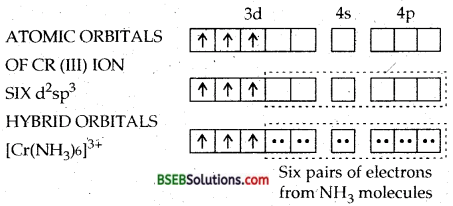The two 3d, one 4s and three 4p orbitals then hybridise to yield six d2sp3 hybrid orbitals pointing towards the six ends of an octahedron. The six ammonia molecules then donate a pair of electrons to each of these vacant orbitals. Consequently, the structure of the complex is octahedral. The presence of three unpaired electrons in the remaining orbitals of Cr (III) makes the complex paramagnetic.

(b) [Fe (CN)6]3- ion. In this complexion, the oxidation no. of iron is + 3.
Fe = [Ar] 3d64s2
Fe = [Ar] 3d5 4s°
The ferric ion must make available six empty orbitals to accomodate six electron pairs from six cyanide ions. This can be achieved by the following hybridisation scheme wherein electrons in d-sub-shell have been paired up as CN ions are strong field ligands.Thus six pairs of electrons from six cyanide ions occupy the six hybridised orbitals of iron (III) ion. At the same time, one unpaired electron remains as such in the three unhybridised orbitals of iron (III) ion. As a result, the complex will show slight paramagnetism corresponding to one unpaired electron. This is actually so. Hence, the complex ion, [Fe(CN)6]3- is paramagnetic and is octahedral.
(c) [Fe (CN)6]4- ion. In this complex ion, the oxidation state of iron is + 2. Let us find out electronic configuration of Fe2+ ion.
Electronic configuration of Fe = [Ar] 3d64s2 Electronic configuration of Fe2+= [Ar] 3d6
The iron (II) ion must make available six empty orbitals to accomodate six pairs of electrons from six cyanide ions. This can be achieved by the following hybridisation scheme wherein electrons in the d-subshell have been paired up as CN ions are strong field ligands.Thus six pairs of electrons from six cyanide ions occupy the six hybridised orbitals of iron (II) ion. At the same time, we find that no orbital which contains unpaired electron. Hence, the ion [Fe (CN)6]4- is diamagnetic. Therefore, [Fe (CN)64- ion is diamagnetic and octahedral.
(d) [CoF6]3- ion-In this complex ion, the oxidation state of cobalt is + 3. Let us find out electronic configuration of Co3+ ion.
Electronic configuration Co = [Ar] 3d74s2
Electronic configuration of Co3+ ion =[Ar] 3d6

The cobalt (III) ion must make available six empty orbitals to accommodate six electron pairs from six fluoride ions. This can be achieved by the following hybridisation scheme where in electrons in the d-subshell have not been paired up as F~ ions are weak field ligands.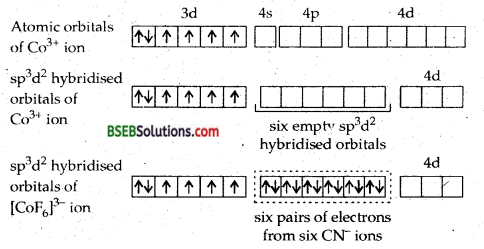Thus six pairs of electrons from six fluoride ions occupy the six hybridised (sp3d2) orbitals of cobalt (III) ion. At the same time, we find that there are four unpaired electrons in the unhybridised d-orbitals of cobalt (III) ion. As. a result, the complex will show paramagnetism corresponding to four unpaired electrons. Hence, the complex ion [CoF6]3- ion is paramagnetic and octahedral.

The complexion [CoF6]3- is outer-orbital complex as d-orbitals of the outer shell have been involved in hybridisation.
(e) [Ni(CO)4]-In this complex, the oxidation state of nickel is zero. The electronic configuration of nickel in the zero oxidation state is [Ar] 3d8,4s2. In order to accomodate 4 electron pairs donated by four carbonyl ligands, the nickel atom must make available a set of four empty orbitals. This is achieved by pushing 4s electrons into 3d orbitals whereby pairing all the 3d electrons. This has been because O is a strong field ligand.
There will be no unpaired electron. Thereafter, nickel atom will undergo sp3 hybridization as shown below :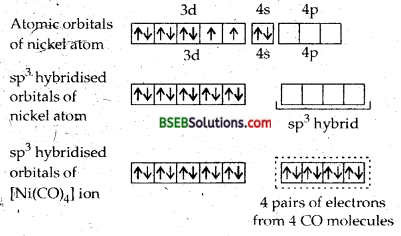Thus four pairs of electrons from four carbon monoxide molecules occupy the four sp3 hybridised orbitals of nickel. Thus we find there is no orbital which contains unpaired electron. Hence, the complex [Ni (CO)4] is diamagnetic. Hence, the complex Ni (CO)4] is diamagnetic and tetrahedral.
(f) [Ni(CN)4]2- Tetracyanonickelate (II) CN being a powerful ligand and will pair up the electrons leaving one d orbital free for hybridization dsp2. The complex will be square planar and diatomic.Question 2.
How will you apply CRYSTAL FIELD THEORY to OCTAHEDRAL and TETRAHEDRAL COMPLEXES? What is meant by STRONG and WEAK-LIiGANDS? What TS CRYSTAL FiELD – TLTFTTNG ENERGY? How does it explain the colour of the complexes?
Crystal Field Theory-The Crystal Field Theory (CFT) was originally proposed for explaining the optical properties of crystalline solids. It was applied to the study of coordination compounds in the 1950s. CFT assumes the ligands to be point charges and the interaction between them and the orbitals in an isolated gaseous metal atom/ion have same energy, Leythey are degenerate. This degeneracy is maintained.

if a spherically symmetrical field of negative charges surrounds the metal atom/ion. However, when this negative field is due to ligands (either anions or the negative ends of dipolar molecules like NH3 and H2O) in a complex, it becomes asymmetrical and the degeneracy of the d orbitals is lifted. It results in split King of the d orbital energies. The pattern of splitting depend upon [the nature of the crystal field. The splitting of d orbital energies and its effects, in fact, form the basis of the crystal field treatment of coordination compounds. We will first consider: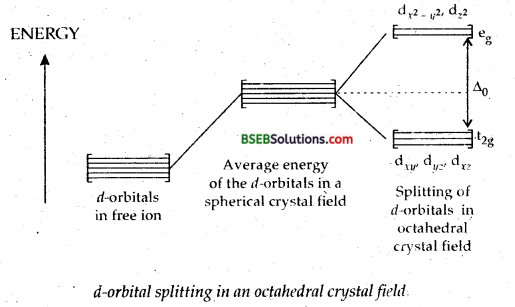(A) Crystal Field Effects in Octahedral Coordination Entities- For convenience, let us assume that the six ligands are positioned symmetrically along the cartesian axes, with the metal atom at the origin. As the ligands approach, first there is an increase in the energy of d orbitals relative to that of the free ion just as would be the case in a spherical field. Next, the orbitals lying along the axes (dZ2 and dx2y2) get repelled more strongly than dxy, dyz and dxz orbitals, which have lobes directed between the axes The dZ and dx2 -y2 orbitals get raised in energy and dxy, dyz, dxz orbitals are lowered in energy relative to the average energy in the spherical crystal field. Thus, the degenerate set of d orbitals get split into two sets: the lower energy orbital set, t2g and the higher energy, eg set. The energy separation is denoted by Δ0 (the subscript o is for octahedral).

Let us now learn about the significance of A0 by considering first the d1 coordination entity. [Ti (H2O)6]3+, formed in aqueous solutions of [Tl3+ (d1) ion. Obviously, the singled electron occupies one of the lower energy t2g orbitals. In d2 and d3 coordination entities, the d electrons occupy the t, orbitals singly in accordance with the Hand’s rule. For d4 ions, two possible patterns of electron distribution arise : (i) the fourth electron may enter an eg orbital (of higher energy) or (ii) it may pair an electron in that L level. The actual configuration adopted is decided by the relative values of Δ0.and P.P represents the energy required for electrons pairing in a single orbital.

If Δ0 is less than P (Δ0 < P), we have the so-called weak field, high spin situation, and the fourth electron enters one of the eg orbitals giving the configuration t32g, e1g. If now a fifth electron is added to a weak field coordination entity, the configuration becomes t32g, e2g When Δ0 < P, we have the strong field, low spin situation, and pairing will occur in the t2g level with the eg level remaining unoccupied in entities of d1 to d6 ions. Calculations show that coordination entities with four to seven d electrons are more stable for strong fields as compared to weak field cases.(B) Crystal Field Effect in Tetrahedral Coordination Entities—In tetrahedral coordination entity formation, the d orbitai splitting is inverted and smaller as compared to the octahedral field splitting. For the same metal, the same ligands and metal-ligand distances, it can be shown that Δ1 = – 4/9Δ0. Consequently, the orbitals splitting energies are not sufficiently large for forcing pairing and configurations are rarely observed.

Important factors which determine the magnitude of Δ the orbital splitting energy, are:
(i) Oxidation state of the metal ion-Generally, the higher the ionic charge on the central metal ion, the greater the value of Δ.

(ii) Nature of the metal ion-For analogous entities within a group, the A value differ. The general trend being 3d < 4d < 5d. Thus while going from C1 of Mo or Co to Rh, the Δ0 value increasingly by – 50%. As a consequence of this, coordination entities of the second and third transition series have a greater tendency to be low spin as compared to the first transition series.

(iii) Geometry of the coordination entity-The Δt, value is only – 50% as large as that of Δ0.
(iv) Nature of the ligand-The ligand can be arranged in the order of increasing field strength.
Colour in Coordination Compounds-Coordination compounds of transition metals have fascinating colour. Colours of transition metal ions in aqueous solution correspond to the presence of their aqua complexes. Further, while aqueous solution of octahedral [Co(H2O)6]2+ is pink, that of tetrahedral [Co Cl]2- is blue.

The colour of aqueous [Ni (H2O)6]2+ turns blue when ammonia is added to it, giving [Ni(NH3)6]3+. Violet [Cr(H2O)6]2+ gives bright blue [Cr(H2O)6]2+ upon reduction. We know that the colour of a substance is due to the absorption of light at a specific wavelength in the visible part of the electromagnetic spectrum (400 to 700 nm) and transmission of reflection of the rest of the wavelength.

An object that absorbs all visible light appears black. Table below gives the relationship of the wavelength absorbed and the colour observed. The mechanism of light absorption in coordination compounds is that photons of appropriate energy can excite the coordination entity from its ground state to an excited state. However, in the case of copper (II) ions in solution, for example, it can be imagined that one of the d-electrons from that t2g set (dxy, dyz, dxz) gets excited to the eg set (dx2– y2, dz2 orbitals). In this case since high energy is transmitted it means that low energy light (red region) is absorbed. For copper (II) ions in aqueous solution, the energy gap Δ0 is relatively small.
By using spectroscopic data for a number of coordination compound having the same metal ion but different ligands, the crystal field splitting for each ligand has been calculated and the spectrochemical series:
I < Br < S2- < SCN < Cl < F OH < C2O42- < O2- < H2O < NCS < py, NH3 < CN < CO established which helps in understanding the nature of bonding and structures of these compounds.

Relationship between the wavelength of light absorbed and the colour observed in some coordination entities
Coordination entity Wavelength of light absorbed (nm) Colour of light absorbed Colour of